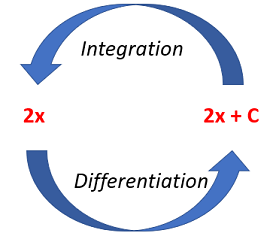# Integrate y with Respect to x

When you integrate y with respect to x, you’re finding the area under the curve when y is plotted against x.

Watch the video for an example:

In symbols, “Integrate y with Respect to x” is written as:

∫ydx

Where:

• ∫ is the integration symbol,
• dx means “with respect to x.”

To solve this type of problem, just place the given function in place of the “y” in ∫ydx, then integrate (using the usual rules of integration).

## How to integrate y with respect to x: Power function ExampleThe rules of integration are opposite to the rules of differentiation. To differentiate a power function, you need to multiply by the original exponent and then reduce the exponent by one (see: General Power Rule). As a formula, that’s:
d/dx (xn) = n x(n – 1).
To integrate a power function, you do the opposite, i.e., increase the exponent by one and then divide by the new exponent, so that:
∫ xn = x(n + 1) / (n + 1) + c.

Example problem: Integrate y = x2 + 1 with respect to x, for x between 0 and 1.
Step 1: Increase the exponent of each term by one, and divide each term by the new exponent.
∫ y dx = [int] (x2 + 1) dx = x3 / 3 + x.

Step 2: Substitute the limits of the integration range for x.
At x = 1, x3 / 3 + x = 4/3
At x = 0, x3 / 3 + x = 0

Step 3: Find the difference between the values (i.e. subtract the values in the previous step).
The value of y integrated with respect to x for x between 0 and 1 is 4/3.

## What if There are No Limits of Integration?

If the example had not given an integration range, the answer would be x3 + x + c.
If you are calculating the indefinite integral of y with respect to x (i.e., if the range of integration is not defined), you need to add an arbitrary constant “+ c” to the end of the equation. This represents the fact that there are a range of possible functions that will differentiate with respect to x to give y, because constant terms disappear under differentiation. Alternatively, a range of integration might be given, in which case, put the given values of x into the integrated expression and find the difference between the two values, as in the example below.

## References

Price, E. (2017). Quiz 15 Solutions. Retrieved September 9, 2013 from: http://www.math.purdue.edu/~price79/SP17/Quiz15Solutions.pdf

CITE THIS AS:
Stephanie Glen. "Integrate y with Respect to x" From StatisticsHowTo.com: Elementary Statistics for the rest of us! https://www.statisticshowto.com/integrals/integrate-y-with-respect-to-x/